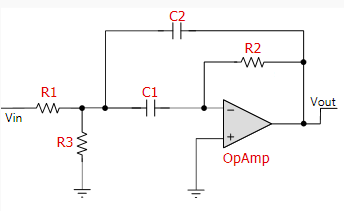# Multi staging filters and impedance considerations

I'm trying to make a MFB Band Pass filter with 80dB attenuation and a bandwidth of around 70hz - 130hz. Each MFB has 40dB attenuation so I want to put two of them and then have two all-pass filters to correct some phase shifts around center frequency. That makes a whopping 4 stage filter. I however have difficulty understanding how I will care about impedances.Now, I care about impedances. Chosing my bandwidth and frequencies isn't much difficult given some practical equations (selecting Q factor, selecting gain, selecting center freq, etc.). My concern however is in staging, if I put these four (2 MFBs then 2 AllPass) in series, while not using each time a follower.

1) Practically speaking, what would be the best way to make sure I end up with an overall gain of two (for example) considering input and output impedances? Is there a way in a simulator like Altium to do it fast? Should I litterally just do it by trial error? What designers normally do.

2) And theoretically speaking, what would I do (purely mathematically speaking on paper). I had as an idea to calculate Zout of the MFB with an AC test voltage source at Vout of the MFB (other sources cancelled), and then using $R_{out} = \frac{V_{out}}{I_{out}}$

I would then do the same for $Z_{in}$ and could then use $V_{in} = \frac{Z_{in\ n}}{Z_{in\ n} + Z_{out\ n-1}}$. Does that make sense or am I completely in the wrong?

I'm just trying to make sense of all this so I don't end up having my signal filtered but attenuated too much because of impedances.

Thanks

I think you should not spend your time and effort for calculating and considering output impedances. The influence of the output impedances (very small due to opamps with feedback) on the overall performance will be negligible if compared with the influence of parts tolerances.

By the way: Are you aware that the FIRST circuit is a 2nd-order lowpass (and NOT a bandpass) ?

Update (Further comment): It is not correct to say that a second-oder bandpass "has 40 dB attenuation". Instead, it approaches a SLOPE of the transfer function (for frequencies far below or far above the center frequecy) of 20dB/dec only. That means: Certainly, there will be a frequency with 40dB attenuation if compared with the mid-frequency. However, it is not clear if this property can fulfill your requirements (this stop frequency is not known yet).

• Hi, could you elaborate on why feedback makes the output impedance low? It's not the first time I recall calculating an output impedance and ending up with zero because the gain is infinite. Is there a general way of proving that feedbacks does that, or is it a kind of "empirical" rule? Does it work for most filters/op amp circuits with feedback? Is there an intuitive way of proving it? (I'm quite good on theory but poor on practice so far). Beside, what do you mean by "parts tolerance"? Feb 10 '17 at 21:04
• Last question: Let`s assume that you need 2.61 kOhms - can you find such a resistor? Most probably, you will use a part with a nominal value of 2.7k - but the actual value is unknown to you. Regarding fedback: Each relevant textbook on opamps will tell you that negative feedback will (a) reduce the the gain and (b) increase the input impedance and (c) reduce the output impedance - all by the same factor: (1-loop gain). Note that for neg. feedback the loop gain itself has a negative sign. If you are allowed to assume an infinite open-loop gain for the opamp, the mentioned factor is infinite.
– LvW
Feb 10 '17 at 21:23
• Yannick, the first circuit is a lowpass (and NOT a bandpass).
– LvW
Feb 11 '17 at 7:40
• Hi, I guess I googled images a little bit in haste, thanks. Component value will not be a huge factor for me because I do not need ultra precise 3db freqs. Just want a good enough noise filtration. I wonder if I should make this another question or not but I'll give it a shot, feel free to answer. If I chose my component values with a kind of "design recipe" like here tinyurl.com/zlolpvb, I care about passband ripple. Should I instead calculate my transfer function with the butterworth approx for low pass and then apply freq transform (to get a butterworth BP filter) Feb 12 '17 at 6:28
• Yannick, the answer primarily depends on the required filter order. If a 2nd-order bandpass can meet your requirements - follow the "design recipe". For a 4th-order filter there are other alternatives. At first - clarify the specification (damping requirements)
– LvW
Feb 12 '17 at 8:11